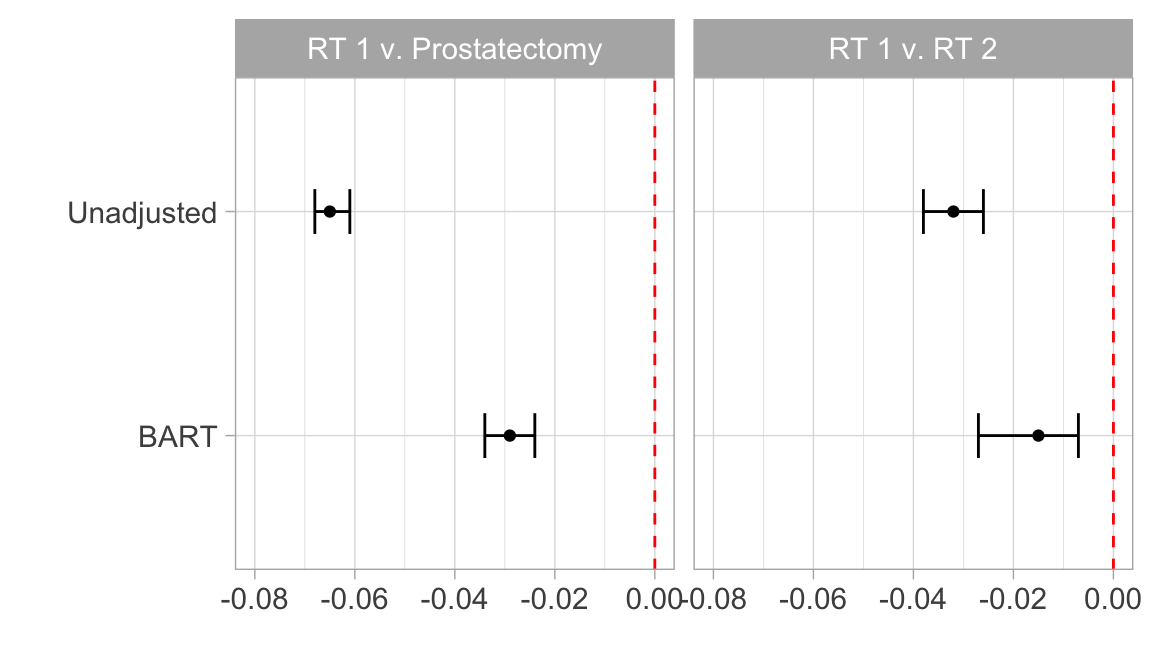# Bayesian additive regression trees for causal inference with multiple treatments

Michael Lopez (with Liangyuan Hu, Chenyang Gu)

## BART with multiple treatments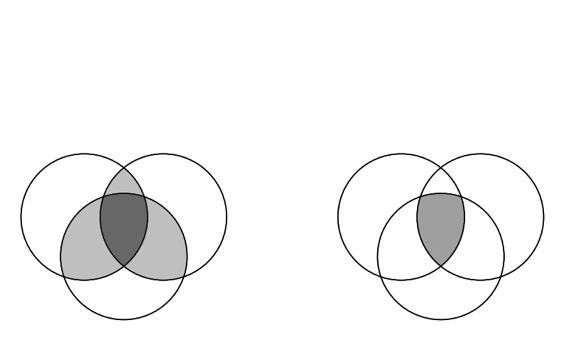Michael Lopez, Liangyuan Hu, Chenyang Gu https://github.com/statsbylopez/ci-bart

## The setting: 3 prostate cancer treatments

Treatment n Death Rate
Prostatectomy 15435 0.094
RT 1 24688 0.029
RT 2 2642 0.061

## The setting: 3 prostate cancer treatments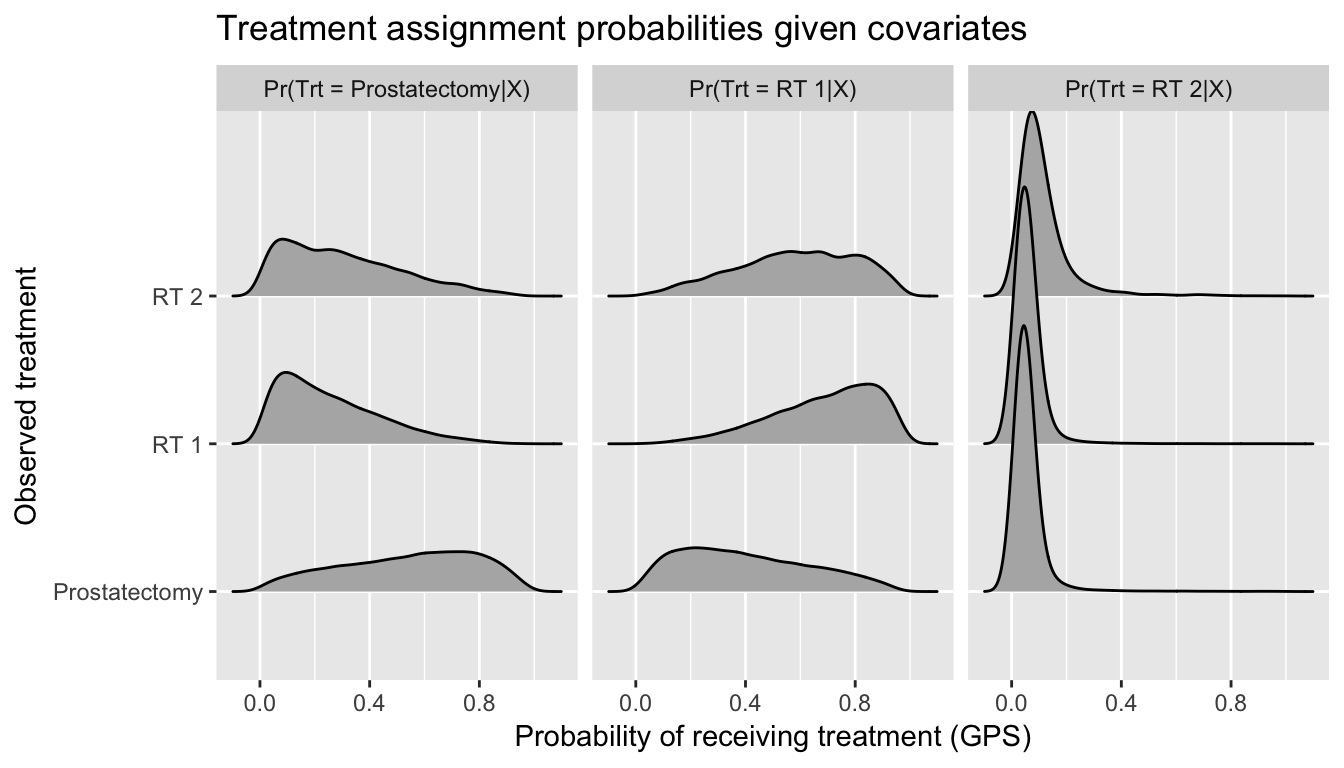## Issue 1: Selection bias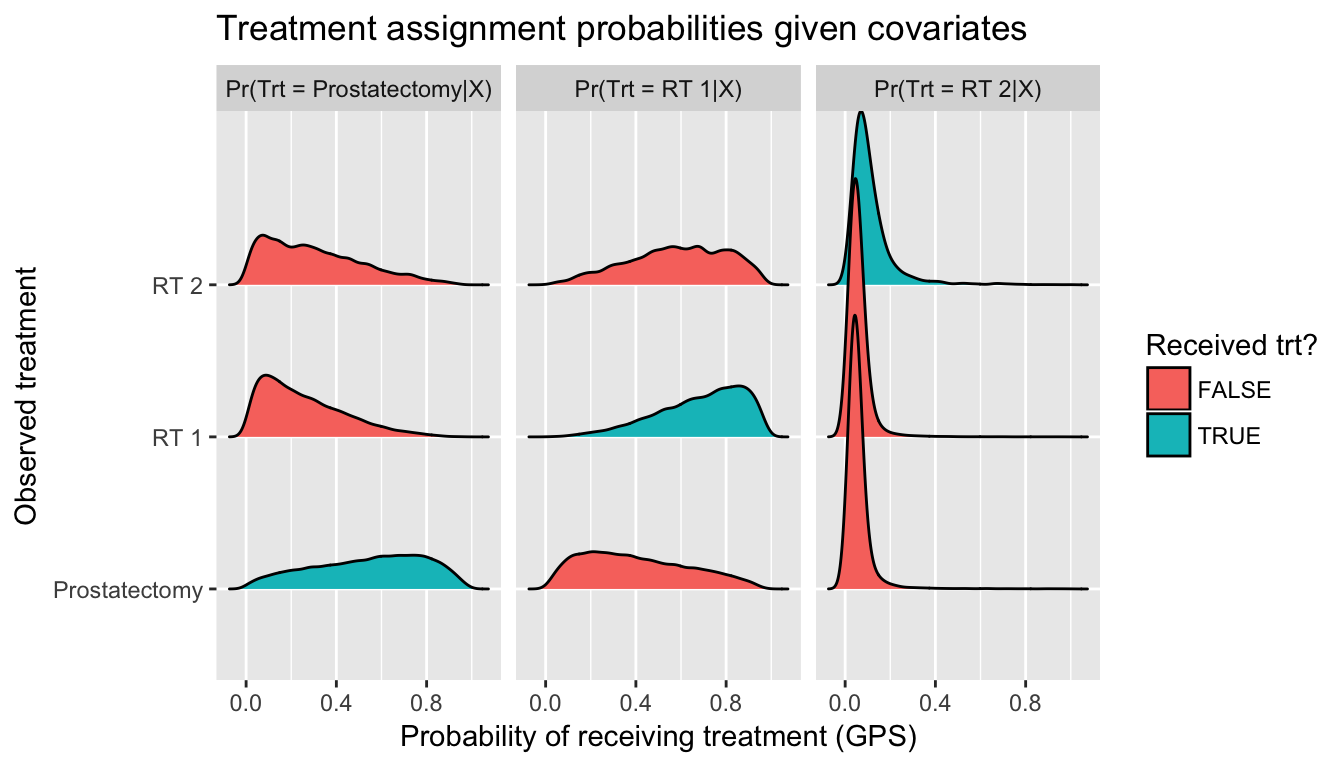## Issue 2: Non-overlapping distributions## Issue 3: Large weights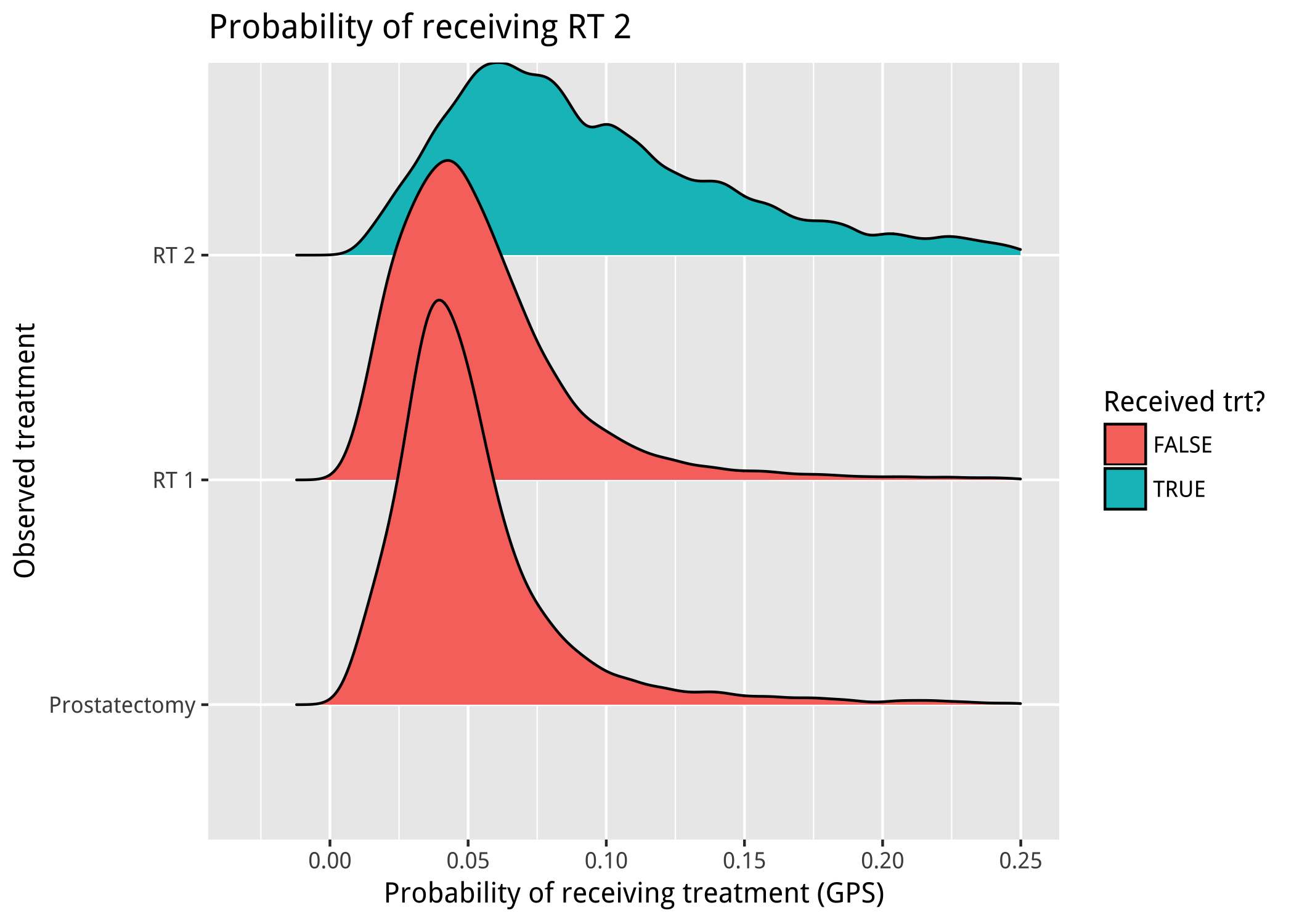## Issue 3: Large weights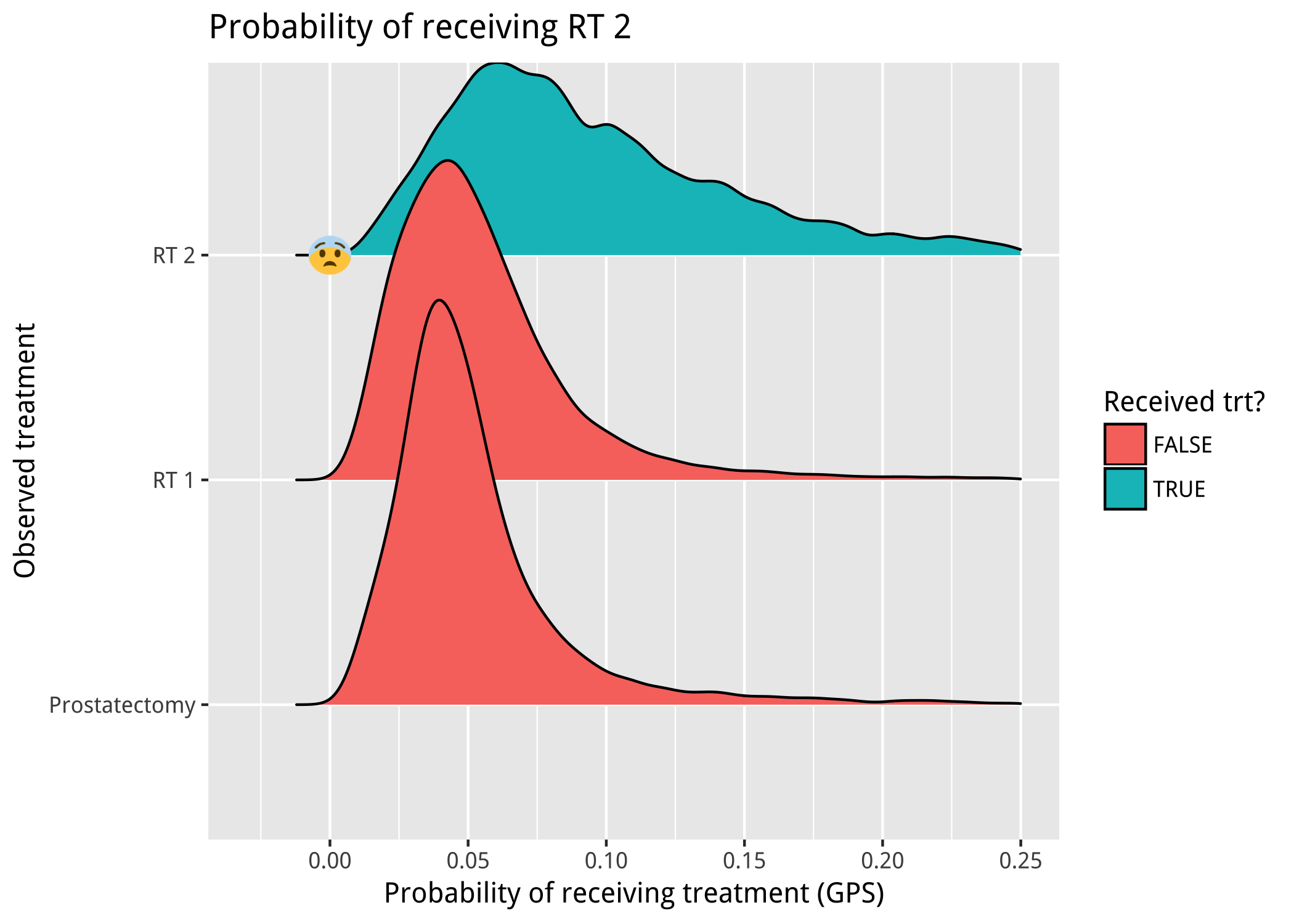## Notation

Consider causal effect of $$A \in \{1, \ldots, Z\}$$ on binary outcome $$Y \in \{0,1\}$$

• $$i = 1, \ldots, n$$ for $$n$$ total subjects
• $$n = n_1 + \ldots + n_Z$$
• $$\{Y_i(1), \ldots, Y_i(Z)\}$$ as potential outcomes for $$i$$
• Covariates $$X_i$$

## Notation

Interest: average treatment effect among treated

## Causal inference with multiple treatments

Why not binary approaches?

## Causal inference with multiple treatments

BART model:

• $$P(Y=1|A=a, X=x) = \Phi(f(a, x))$$
• $$f(a,x)$$ appromated using sum of trees
• $$ATT$$’s estimated using counterfactuals
• $$ATT_{1|1, a} = \frac{1}{n_1} \sum_{i:A_i = 1}^{n} \Phi(f(1, x_i)) - \Phi(f(a, x_i))$$
• $$ATT_{1|2, a} = \frac{1}{n_1} \sum_{i:A_i = 1}^{n} \Phi(f(2, x_i)) - \Phi(f(a, x_i))$$

Why BART for causal inference? see Hill, 2012

• Flexibly models response surface ✔️
• Large number of continuous and categorical predictors ✔️
• No ambiguity with respect to balance assessment ✔️
• Accessibility ✔️
• Accuracy ✔️

Why BART for multiple treatments?

• Coherent posterior intervals ❓
• Heterogenous treatment effects ❓
• Accessibility ❓
• Accuracy ❓

## Simulation study

6 factorial design using dbarts package in R

• Ratio of $$n_1$$ : $$n_2$$ : $$n_3$$
• $$n$$
• No. predictors
• $$P(A|X)$$
• Predictor strength alignment
• Response surfaces (parallel?)

## Simulation results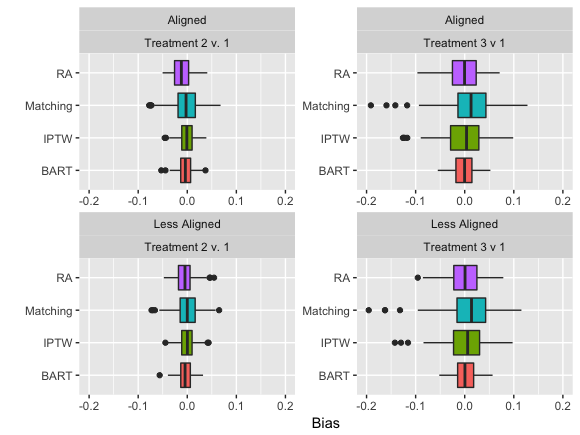## 3 prostate cancer treatments

ATT’s: generalizable to population receiving RT 1